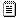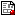[Previous] [Next] [Up] [Top]

# Assignment 3

Multivariate Data Analysis
Psychology 6140

1. Matrix algebra: Green & Carroll, Chapter 2, 2.7 thru end; Green & Carroll 3.1-3.4 (Morrison, 2.4-2.6)
2. Introduction to RStudio and a 4-page R Reference Card
3. Introduction to SAS V8 for Windows Sections 1-3 give an overview.
4. SAS/IML Introduction& handouts; also a handy SAS IML Reference Card

## Supplements

In R: vignette("linear-equations", package="matlib)"
demonstrates illustrates some ideas behind solving systems of linear equations in two and three unknowns
Systems of Linear Equations
A brief tutorial
Elementary row operations
A brief tutorial
imlmat1.sasBasic matrix definitions
imlmat2.sasMatrix arithmetic: +, -, # (elementwise operations)
imlmat4.sasProperties of matrix multiplication

## Problems (due: next week)

From the Matrix Algebra Problems (MAP) handout: You can do these by hand, but you might try using R or SAS/IML to do the following problems from Green and Carroll:
• Chap 2, Problem 12
• Chap 2, Problem 15. GC-2-15.csv contains the small data set